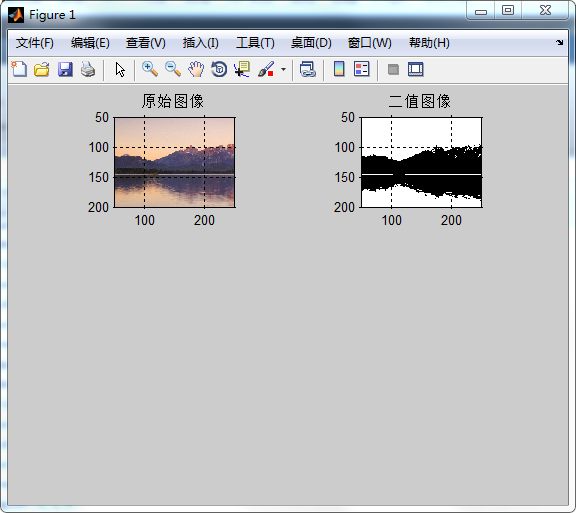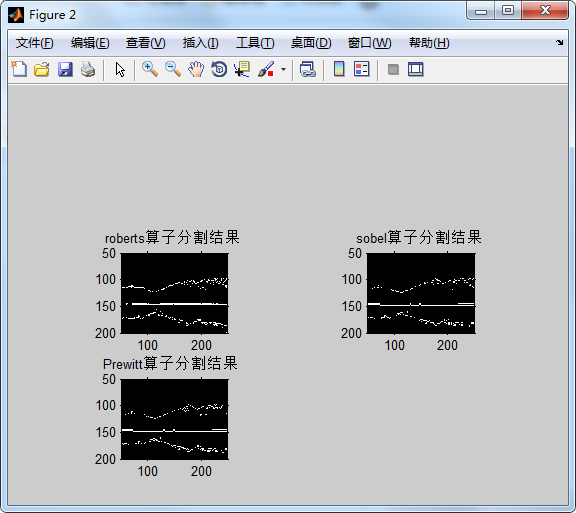2015-05-13 11:44:59 buaalei 阅读数 7159
• ###### Kaggle 神器：XGBoost 从基础到实战

39405 人正在学习 去看看 AI100讲师Prewitt在X, Y方向上梯度算子分别为：opencv Sobel算子函数：

void Sobel(InputArray src, OutputArray dst, int ddepth, int dx, int dy, int ksize=3, double scale=1, double delta=0,int borderType=BORDER_DEFAULT )

src – 输入图像。
dst – 输出图像，与输入图像同样大小，拥有同样个数的通道。
ddepth –

src.depth() = CV_8U, ddepth = -1/CV_16S/CV_32F/CV_64F
src.depth() = CV_16U/CV_16S, ddepth = -1/CV_32F/CV_64F
src.depth() = CV_32F, ddepth = -1/CV_32F/CV_64F
src.depth() = CV_64F, ddepth = -1/CV_64F

xorder – x方向导数运算参数。
yorder – y方向导数运算参数。
ksize – Sobel内核的大小，可以是：1，3，5，7。
scale – 可选的缩放导数的比例常数。
delta – 可选的增量常数被叠加到导数中。
borderType – 用于判断图像边界的模式。

//在x方向求图像近似导数

Sobel( src_gray, grad_x, ddepth, 1, 0, 3, scale, delta, BORDER_DEFAULT );
//在y方向求图像近似导数
Sobel( src_gray, grad_y, ddepth, 0, 1, 3, scale, delta, BORDER_DEFAULT );

convertScaleAbs函数

void convertScaleAbs(InputArray src, OutputArray dst, double alpha=1, double beta=0)

src – 输入数组。
dst – 输出数组。
alpha – 可选缩放比例常数。
beta – 可选叠加到结果的常数。

void addWeighted(InputArray src1, double alpha, InputArray src2, double beta, double gamma, OutputArray dst, intdtype=-1)

src1 – 第一个输入数组。
alpha – 第一个数组的加权系数。
src2 – 第二个输入数组，必须和第一个数组拥有相同的大小和通道。
beta – 第二个数组的加权系数。
dst – 输出数组，和第一个数组拥有相同的大小和通道。
gamma – 对所有和的叠加的常量。
dtype – 输出数组中的可选的深度，当两个数组具有相同的深度，此系数可设为-1，意义等同于选择与第一个数组相同的深度。

dst = src1*alpha + src2*beta + gamma;

/////////////////////////// Sobe l////////////////////////////////////
//Scharr( src_gray, grad_x, ddepth, 1, 0, scale, delta, BORDER_DEFAULT );
//Calculates the first, second, third, or mixed image derivatives using an extended Sobel operator.
Sobel( src_gray, grad_x, ddepth, 1, 0, 3, scale, delta, BORDER_DEFAULT );
//Scharr( src_gray, grad_y, ddepth, 0, 1, scale, delta, BORDER_DEFAULT );
Sobel( src_gray, grad_y, ddepth, 0, 1, 3, scale, delta, BORDER_DEFAULT );

2019-04-06 19:11:31 Small_Munich 阅读数 244
• ###### Kaggle 神器：XGBoost 从基础到实战

39405 人正在学习 去看看 AI100讲师
##### 图像梯度算子简介

相信只要是懂些图像处理的知识，都知道图像梯度的含义。不知道是否考虑过为什么图像梯度如此广泛认知与使用？为什么不使用图像纹理、图像色彩、图像相位等等，在这里我并不是说上述除了图像梯度之外，其它的图像信息不重要，我只是想说图像梯度最为广泛的被研究与使用。下面我们来看一幅图像：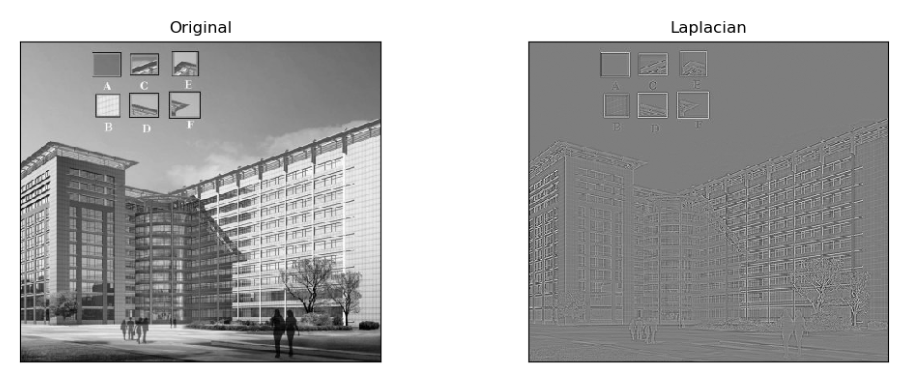从上图坐标为灰度图像，右边为Laplacian算子提取的图像梯度图；现在我们来看一下左边图片中的A、B、C、D、E、F区域，你可以通过这些子区域发现处于图像中的哪个位置吗？通过人眼观察可以轻易得出结果：E、F最容易分辨，C、D能够确定更小的范围区域，A、B最难分辨在哪个范围。OK，让我们来看右边的梯度算子，可以发现：E、F小区域在梯度图像上较为完整的保留下来，C、D保留边缘曲线，A、B已经完全发现不出什么明显的特征。

通过上述一个简单的观察，你会发现：E、F是角点区域(梯度各个方向变化最为明显)，C、D为边缘区域(梯度垂直于边缘方向最为明显)、A、B为平坦区域(梯度各个方向变化都不明显)。这不就是后面做特征点检测的信息丰富度准则，角点最为稳定、边缘检测等特征。图像梯度提取最为简单直接，能够有效的描述图像的原始状态，因此发展一系列的图像梯度算子：Roberts、Prewitt、Sobel、Laplacian、Scharr等。下面介绍一下比较经常使用的梯度提取算子卷积核函数：

Sobel算子：

$W_{sobelx}= \left[ \begin{matrix} w_{00} & w_{01} & w_{02} \\ w_{10} & w_{11} & w_{12} \\ w_{20} & w_{21} & w_{22} \end{matrix} \right] = \left[ \begin{matrix} -1 & 0 & +1 \\ -2 & 0 & +2 \\ -1 & 0 & +1 \end{matrix} \right]$

$W_{sobely}= \left[ \begin{matrix} w_{00} & w_{01} & w_{02} \\ w_{10} & w_{11} & w_{12} \\ w_{20} & w_{21} & w_{22} \end{matrix} \right] = \left[ \begin{matrix} -1 & -2 & -1 \\ 0 & 0 & 0 \\ +1 & +2 & +1 \end{matrix} \right]$

$G_{image}=\sqrt{G^{2}_x+G^{2}_y}\tag{1}$

参数解释：$G_{image}$为Sobel算子提取的梯度图像，$G_x$$W_{sobelx}$卷积核与原始图像卷积提取X方向的结果，$G_y$$W_{sobely}$卷积核与原始图像卷积提取$Y$方向的结果。

Scharr算子：

$W_{scharrx}= \left[ \begin{matrix} w_{00} & w_{01} & w_{02} \\ w_{10} & w_{11} & w_{12} \\ w_{20} & w_{21} & w_{22} \end{matrix} \right] = \left[ \begin{matrix} -3 & 0 & +3 \\ -10 & 0 & +10 \\ -3 & 0 & +3 \end{matrix} \right]$

$W_{scharry}= \left[ \begin{matrix} w_{00} & w_{01} & w_{02} \\ w_{10} & w_{11} & w_{12} \\ w_{20} & w_{21} & w_{22} \end{matrix} \right] = \left[ \begin{matrix} -3 & -10 & -3 \\ 0 & 0 & 0 \\ +3 & +10 & +3 \end{matrix} \right]$

Scharr算子与Sobel算子原理一致，只是改进版，效果要比Sobel好些。

Laplacian算子：

拉普拉斯算子是最广为人知的算子，完全的尺度不变性，依赖二阶导数能够提取稳定的特征点，著名的差分高斯近似加速等等。其提取图像特征的核函数为：

$W_{laplacian}= \left[ \begin{matrix} w_{00} & w_{01} & w_{02} \\ w_{10} & w_{11} & w_{12} \\ w_{20} & w_{21} & w_{22} \end{matrix} \right] = \left[ \begin{matrix} 0 & 1 & 0 \\ 1 & -4 & 1 \\ 0 & 1 & 0 \end{matrix} \right]$

利用卷积核与图像进行卷积运算，以此来提取图像梯度。关于卷积操作具体解释可以参考图像滤波算法系列实战总结之一博文开头卷积示例图片，抱歉暂无找到引用何处，发现后会添加上。

##### OpenCV-Python代码实践
import cv2
import numpy as np
from matplotlib import pyplot as plt

laplacian = cv2.Laplacian(img, cv2.CV_64F)
sobelx = cv2.Sobel(img, cv2.CV_64F, 1, 0, ksize=3)
sobely = cv2.Sobel(img, cv2.CV_64F, 0, 1, ksize=3)

sobelxy = np.sqrt(sobelx*sobelx + sobely*sobely)

scharrx = cv2.Sobel(img, cv2.CV_64F, 1, 0, ksize=-1)
scharry = cv2.Sobel(img, cv2.CV_64F, 0, 1, ksize=-1)

scharrxy = np.sqrt(scharrx*scharrx + scharry*scharry)

plt.subplot(2, 4, 1), plt.imshow(img, cmap='gray')
plt.title('Original'), plt.xticks([]), plt.yticks([])

plt.subplot(2, 4, 2), plt.imshow(laplacian, cmap='gray')
plt.title('Laplacian'), plt.xticks([]), plt.yticks([])

plt.subplot(2, 4, 3), plt.imshow(sobelx, cmap='gray')
plt.title('Sobel X'), plt.xticks([]), plt.yticks([])

plt.subplot(2, 4, 4), plt.imshow(sobely, cmap='gray')
plt.title('Sobel Y'), plt.xticks([]), plt.yticks([])

plt.subplot(2, 4, 5), plt.imshow(scharrx, cmap='gray')
plt.title('Scharr X'), plt.xticks([]), plt.yticks([])

plt.subplot(2, 4, 6), plt.imshow(scharry, cmap='gray')
plt.title('Scharr Y'), plt.xticks([]), plt.yticks([])

plt.subplot(2, 4, 7), plt.imshow(sobelxy, cmap='gray')
plt.title('Sobel XY'), plt.xticks([]), plt.yticks([])

plt.subplot(2, 4, 8), plt.imshow(scharrxy, cmap='gray')
plt.title('Scharr XY'), plt.xticks([]), plt.yticks([])

plt.show()

##### 实验结果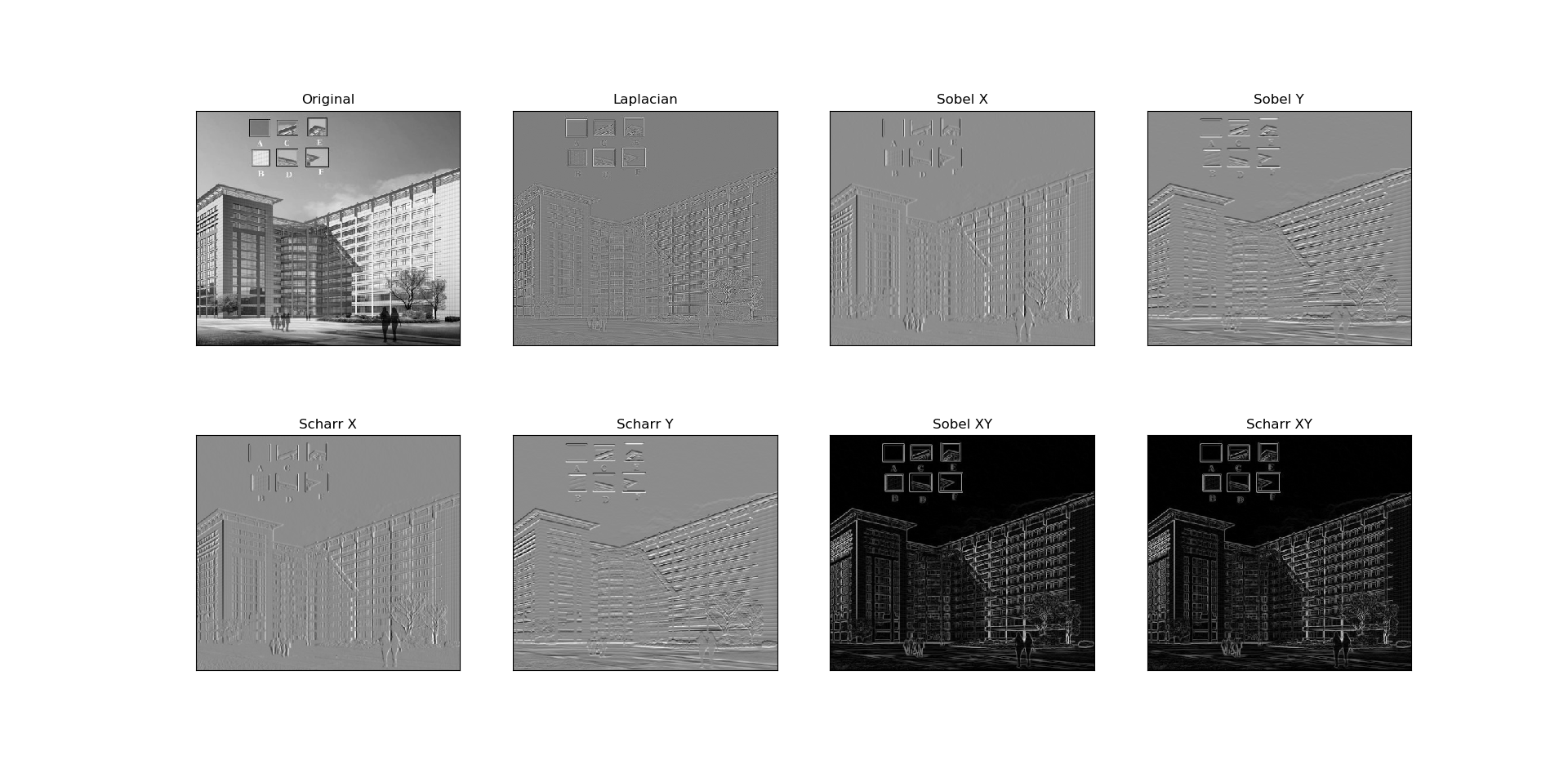##### 参考

2019-04-01 18:27:18 xzc1234__ 阅读数 193
• ###### Kaggle 神器：XGBoost 从基础到实战

39405 人正在学习 去看看 AI100讲师

# 一、图像梯度算子:

1.概念简介（部分引自百度百科）：

Sobel算子：用来进行计算一阶、二阶或混合图像差分。通过对图像用相应的内核进行卷积操作来计算图像差分；由于该算子不进行图像尺度变换，所以和输入图像(数组)相比，输出图像(数组)的元素通常具有更大的绝对数值（即像素的位深）。为防止溢出，当输入图像是 8 位的，要求输出图像是 16 位的。所有输入和输出图像都必须是单通道的，并且具有相同的图像尺寸或者ROI尺寸。

Laplacian算子：主要用来计算图像的二阶微分算子。

2.需用函数：
cv2.Sobel(src,ddepth,dx,dy[,dst[,ksize[,scale[,delta[,borderType]]]]])→dst
5个常用参数分别代表：原图像、图像颜色深度即数据类型（一般用cv2.CV_64F,也可用-1使其数据类型与原图像相同即为np.uint8）、x和y方向上的差分阶数、soble核的大小（必须为1、3、5、7中的一个）

cv2.Sobel(img,cv2.CV_64F,1,0,ksize=3) 仅在x方向上求一阶导数，最高可求二阶
cv2.Sobel(img,cv2.CV_64F,0,1,ksize=3) 仅在y方向上求一阶导数，最高可求二阶

cv2.Scharr(src,ddepth,dx,dy[,dst[,scale[,delta[,borderType]]]]])→dst

cv2.Laplacian(src,ddepth,dx,dy[,dst[,ksize[,scale[,delta[,borderType]]]]])→dst

cv2.convertScaleAbs(src[,dst[,alpha[,beta]]])→dst
x先将原图像的全部值进行绝对值操作，然后将原图像转换为unsigned int8类型

3.代码实现如下：

import cv2
import numpy as np
#只能对单通道图像进行处理，因此以灰度格式读取该图片，第二个参数也可为0

#两种选择输出图像数组数据深度方式来生成目标图像

sobel_x=cv2.Sobel(img,cv2.CV_8U,1,0)
sobel_y=cv2.Sobel(img,cv2.CV_8U,0,1)
# 直接使用unsigned int8类型的图像矩阵，这将会导致求方向导数后的某些负值或超过255的值损失
scharr_x=cv2.Sobel(img,cv2.CV_8U,1,0,ksize=-1)
scharr_y=cv2.Sobel(img,cv2.CV_8U,0,1,ksize=-1)
laplacian=cv2.Laplacian(img,cv2.CV_8U)  #求图像二阶导数

sobel_x=cv2.Sobel(img,cv2.CV_64F,1,0)
sobel_y=cv2.Sobel(img,cv2.CV_64F,0,1)
# 直接使用float32类型的图像矩阵，不会使输出图像某些值的损失，但由于cv2.imshow()函数不支持输出
# float32的图像，因此需要通过cv2.convertScaleAbs()改变输出图像为uint8类型的
scharr_x=cv2.Sobel(img,cv2.CV_64F,1,0,ksize=-1)
scharr_y=cv2.Sobel(img,cv2.CV_64F,0,1,ksize=-1)
laplacian=cv2.Laplacian(img,cv2.CV_64F)
cv2.convertScaleAbs(sobel_x)
cv2.convertScaleAbs(sobel_y)
cv2.convertScaleAbs(scharr_x)
cv2.convertScaleAbs(scharr_x)
cv2.convertScaleAbs(laplacian)
#使用后者这种方法会发现比前者的线条和轮廓更加具体
#还可使用numpy模块中的方法实现输出图像类型的转变
#sobel_x=np.absolute(sobel_x)，sobel_x=np.array(sobel_x,np.uint8)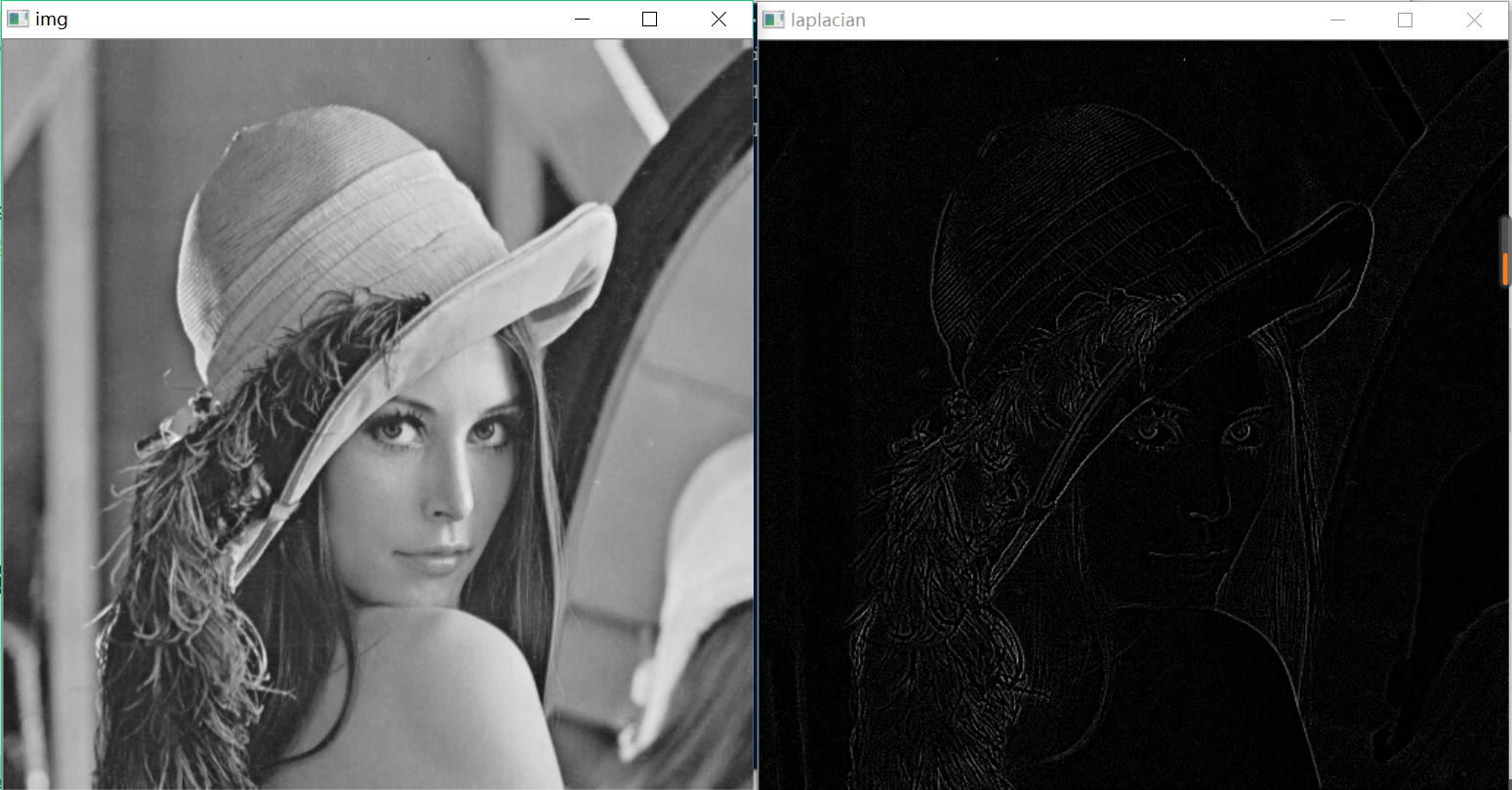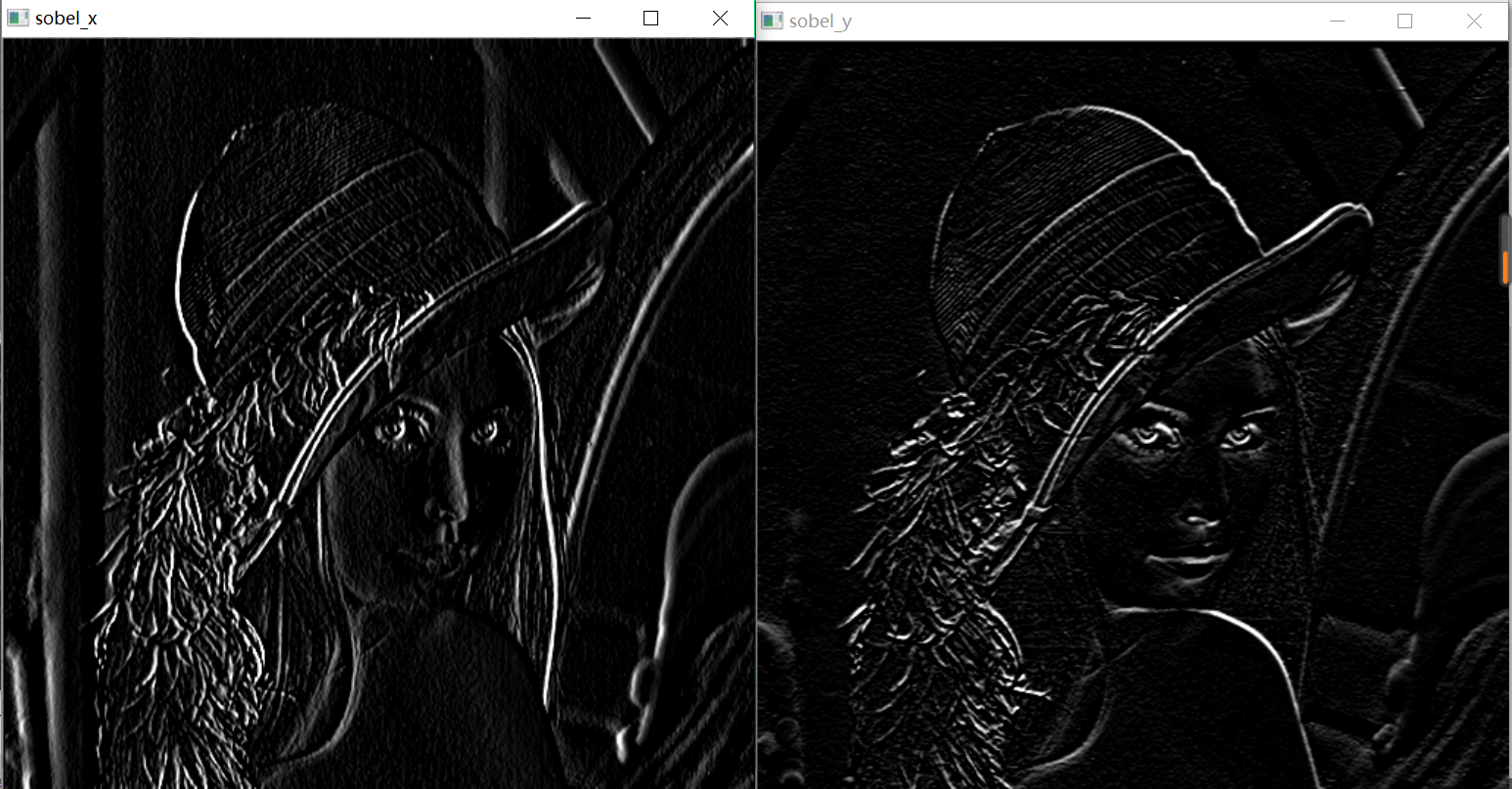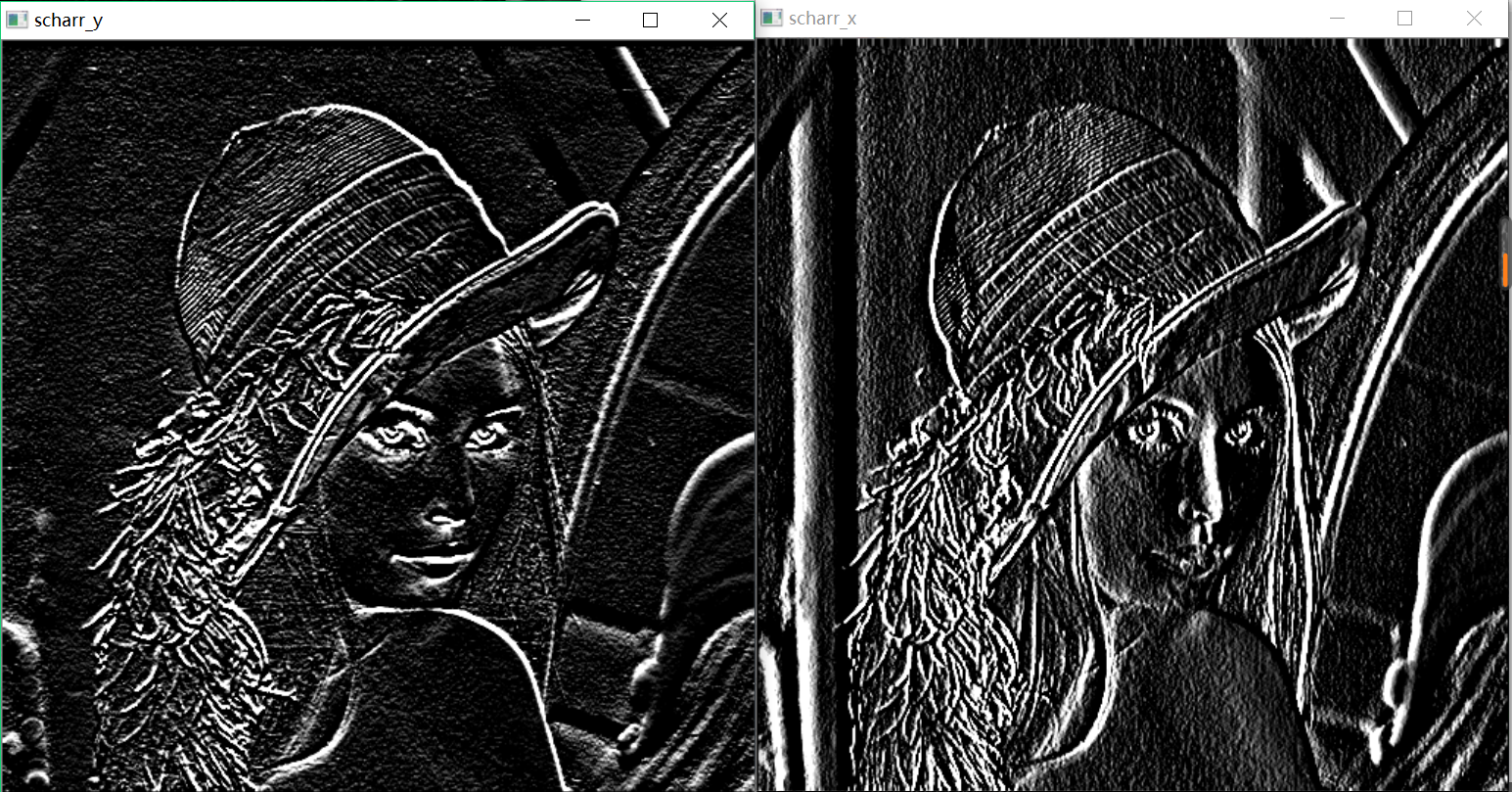# 二、Canny边缘检测（一个多级边缘检测算法）：

1.边缘检测原理简介：（大部分引自百度百科）

(1). 应用高斯滤波来平滑图像。目的是去除噪声，由于噪声对边缘检测影响很大，因此需要先进行平滑操作去除部分噪声
(2).寻找图像的梯度强度。Canny算法的基本思想是寻找一幅图像中灰度强度变化最强的位置。所谓变化最强，即指梯度方向。平滑后的图像中每个像素点的梯度可以由Sobel算子来获得。在变化剧烈的地方（边界处），将获得较大的梯度度量值G，对应的颜色为白色。然而，这些边界通常非常粗，难以标定边界的真正位置。为了做到这一点（需进行非极大值抑制），还必须存储梯度方向，因此在这一步会存数两块数据，分别是梯度的强度信息和梯度的方向信息。
(3).进行非最大值抑制，使边界线更细，找到边界的真正位置即使模糊的边界变得清晰。通俗的讲，就是保留了每个像素点上梯度强度的极大值，而删掉其他的值。对于每个像素点，进行如下操作：
a) 将其梯度方向近似为以下值中的一个（0,45,90,135,180,225,270,315）（即上下左右和45度方向）
b) 比较该像素点和其梯度方向正负方向相邻像素点的梯度强度，如果该像素点梯度强度最大则保留，否则抑制（删除，即置为0），边界处的梯度方向总是指向垂直于边界的方向，即最后会保留一条边界处最亮的一条细线。
(4).应用双阈值的方法来确定可能的边界。高斯平滑后如还有少量噪声，这些噪声点会影响第二步和第三步的边界确定，因此需通过该步骤更全面的确定边界。Canny算法中应用双阈值方法即设定一个阈值上界和阈值下界，图像中的像素点如果大于阈值上界则认为必然是边界（称为强边界），小于阈值下界则认为必然不是边界，两者之间的则认为是候选项（称为弱边界）。
(5).利用滞后技术来跟踪边界。和强边界相连的弱边界认为是边界，其他的弱边界则被抑制。
2.函数简介；
OpenCV使用一个函数和多个参数来完成以上五个步骤

3.代码实现：

import cv2
edges=cv2.Canny(img,100,200)
cv2.imshow("img",img)
cv2.imshow("edges",edeges)
cv2.waitKey(0)
cv2.destroyAllWindows()2018-11-19 14:54:15 qq_25729757 阅读数 5608
• ###### Kaggle 神器：XGBoost 从基础到实战

39405 人正在学习 去看看 AI100讲师

#图像边缘提取——
##梯度算子、Roberts算子、prewitt算子、Sobel算子、Kirsch算子、LOG算子的matlab实现

matlab代码

clear;
T=20;%阈值
[m,n]=size(f);
%------梯度法-------
f_g=zeros(m,n);
for i=2:m-1
for j=2:n-1
f_g(i,j)=abs(f(i+1,j)-f(i,j))+abs(f(i,j+1)-f(i,j));
if f_g(i,j)<T
f_g(i,j)=0;
else
f_g(i,j)=255;
end
end
end
figure(1);
subplot(2,3,1);imshow(uint8(f_g));title('梯度法');

%------roberts算子-------
f_r=zeros(m,n);
for i=2:m-1
for j=2:n-1
f_r(i,j)=abs(f(i+1,j+1)-f(i,j))+abs(f(i,j+1)-f(i+1,j));
if f_r(i,j)<T
f_r(i,j)=0;
else
f_r(i,j)=255;
end
end
end
%f_r=imbinarize(imfilter(f,r),T);
subplot(2,3,2);imshow(uint8(f_r));title('Roberts算子');

%------prewitt算子-------
f_p=zeros(m,n);
for i=2:m-1
for j=2:n-1
f_p(i,j)=abs(f(i-1,j-1)+f(i,j-1)+f(i+1,j-1)-f(i-1,j+1)-f(i,j+1)-f(i+1,j+1))+abs(f(i+1,j-1)+f(i+1,j)+f(i+1,j+1)-f(i-1,j-1)-f(i-1,j)-f(i-1,j+1));
if f_p(i,j)<15
f_p(i,j)=0;
else
f_p(i,j)=255;
end
end
end
subplot(2,3,3);imshow(uint8(f_p));title('Prewitt算子');

%------sobel算子-------
f_s=zeros(m,n);
for i=2:m-1
for j=2:n-1
f_s(i,j)=abs(f(i-1,j-1)+2*f(i,j-1)+f(i+1,j-1)-f(i-1,j+1)-2*f(i,j+1)-f(i+1,j+1))+abs(f(i+1,j-1)+2*f(i+1,j)+f(i+1,j+1)-f(i-1,j-1)-2*f(i-1,j)-f(i-1,j+1));
if f_s(i,j)<T
f_s(i,j)=0;
else
f_s(i,j)=255;
end
end
end
subplot(2,3,4);imshow(uint8(f_s));title('Sobel算子');

%------krisch算子-------
k(:,:,1)=[-3,-3,-3;
-3,0,5;
-3,5,5];
k(:,:,2)=[-3,-3,5;
-3,0,5;
-3,-3,5];
k(:,:,3)=[-3,5,5;
-3,0,5;
-3,-3,-3];
k(:,:,4)=[-3,-3,-3;
-3,0,-3;
5,5,5];
k(:,:,5)=[5,5,5;
-3,0,-3;
-3,-3,-3];
k(:,:,6)=[-3,-3,-3;
5,0,-3;
5,5,-3];
k(:,:,7)=[5,-3,-3;
5,0,-3;
5,-3,-3];
k(:,:,8)=[5,5,-3;
5,0,-3;
-3,-3,-3];
kk=zeros(size(f));
I=double(f);
for i=1:8
f_k(:,:,i)=conv2(I,k(:,:,i),'same');
kk=max(kk,f_k(:,:,i));
end
f_kk=imbinarize(kk,600);
subplot(2,3,5);imshow(f_kk);title('Krisch算子');

%------LoG算子-------
log1=[0 0 -1 0 0;
0 -1 -2 -1 0;
-1 -2 16 -2 -1;
0 -1 -2 -1 0;
0 0 -1 0 0];

f_l=conv2(f,log1,'same');
f_ll=imbinarize(abs(f_l),300);
subplot(2,3,6);imshow(f_ll);title('LoG算子');2018-11-20 17:17:43 dyq1995 阅读数 3726
• ###### Kaggle 神器：XGBoost 从基础到实战

39405 人正在学习 去看看 AI100讲师

下面简要介绍一下不同的梯度算子对于图像处理的区别：

1、首先打开MATLAB软件，在主界面的编辑器中写入下列代码：

subplot(3,2,1);
imshow(I);
title('原始图像');
axis([50,250,50,200]);
grid on;                  %显示网格线
axis on;                  %显示坐标系
I1=im2bw(I);
subplot(3,2,2);
imshow(I1);
title('二值图像');
axis([50,250,50,200]);
grid on;                  %显示网格线
axis on;                  %显示坐标系
I2=edge(I1,'roberts');
figure;
subplot(3,2,3);
imshow(I2);
title('roberts算子分割结果');
axis([50,250,50,200]);
grid on;                  %显示网格线
axis on;                  %显示坐标系
I3=edge(I1,'sobel');
subplot(3,2,4);
imshow(I3);
title('sobel算子分割结果');
axis([50,250,50,200]);
grid on;                  %显示网格线
axis on;                  %显示坐标系
I4=edge(I1,'Prewitt');
subplot(3,2,5);
imshow(I4);
title('Prewitt算子分割结果 ');
axis([50,250,50,200]);
grid on;                  %显示网格线
axis on;                  %显示坐标系

2、命名保存好之后，点击运行按钮，出现如下所示结果：